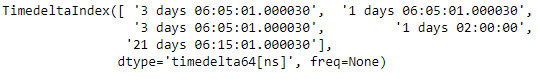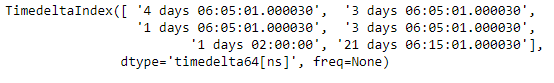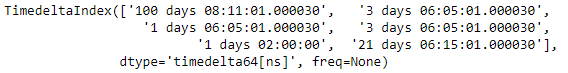Skip to content
Related Articles
Python | Pandas TimedeltaIndex.insert
• Last Updated : 28 Dec, 2018

Python is a great language for doing data analysis, primarily because of the fantastic ecosystem of data-centric python packages. Pandas is one of those packages and makes importing and analyzing data much easier.

Pandas` TimedeltaIndex.insert()` function return the underlying data as an ndarray of the given TimedeltaIndex object.

Syntax : TimedeltaIndex.insert(loc, item)

Parameters :
loc : int
item : if not either a Python datetime or a numpy integer-like, returned Index dtype will be object rather than datetime.

Return : new_index : Index

Example #1: Use `TimedeltaIndex.insert()` function is used to insert new values in the TimedeltaIndex objects. It returns a new object having the new element inserted at the desired position.

 `# importing pandas as pd``import` `pandas as pd`` ` `# Create the TimedeltaIndex object``tidx ``=` `pd.TimedeltaIndex(data ``=``[``'3 days 06:05:01.000030'``, ``'1 days 06:05:01.000030'``,``                                ``'3 days 06:05:01.000030'``, ``'1 days 02:00:00'``,``                                                ``'21 days 06:15:01.000030'``])`` ` `# Print the TimedeltaIndex object``print``(tidx)`

Output :Now we will use the `TimedeltaIndex.insert()` function to insert a value at the 0th position

 `# insert the passed value at 0th position``tidx.insert(loc ``=` `0``, item ``=``'4 days 06:05:01.000030'``)`

Output :As we can see in the output, the `TimedeltaIndex.insert()` function has inserted the passed value at the desired location in tidx object.

Example #2: Use `TimedeltaIndex.insert()` function is used to insert new values in the TimedeltaIndex objects. It returns a new object having the new element inserted at the desired position.

 `# importing pandas as pd``import` `pandas as pd`` ` `# Create the TimedeltaIndex object``tidx ``=` `pd.TimedeltaIndex(data ``=``[``'06:05:01.000030'``, ``'3 days 06:05:01.000030'``, ``                                ``'22 day 2 min 3us 10ns'``, ``'+23:59:59.999999'``, ``                             ``'13 days 06:05:01.000030'``, ``'+12:19:59.999999'``])`` ` `# Print the TimedeltaIndex object``print``(tidx)`

Output :Now we will use the `TimedeltaIndex.insert()` function to insert a value at the 0th position

 `# insert the passed value at 0th position``tidx.insert(loc ``=` `0``, item ``=``'100 days 08:11:01.000030'``)`

Output :As we can see in the output, the `TimedeltaIndex.insert()` function has inserted the passed value at the desired location in tidx object.

Attention geek! Strengthen your foundations with the Python Programming Foundation Course and learn the basics.

To begin with, your interview preparations Enhance your Data Structures concepts with the Python DS Course. And to begin with your Machine Learning Journey, join the Machine Learning – Basic Level Course

My Personal Notes arrow_drop_up